# 20 Daily Life Math Facts that will blow your mind

Most of us assume that Mathematics is boring. Plenty of things in the subject are uninteresting. The one who hate the subject hardly cares that negative times a negative is always positive whereas the area of a circle is πr². Well, it always crosses most of our minds. When examinations approach, we spend days and nights to solve the math puzzlers. It’s no denying fact that for some maths is no less than a boring subject while for others it’s simply a lifeline.

Mathematics is a part and parcel of life and undoubtedly, there are a plethora of daily life math facts that will blow your mind. If you are hunting for some intriguing mathematical facts, then the following facts are just enough to make you ponder how it is possible:

# Daily Life Math Facts

Birthday Paradox: It has a complicated maths behind it. It says that if a room is filled with 23 people, it’s 50 percent sure that two people would share a common birthday. It appears counter-intuitive due to the probability of having same birthday is only 1/365.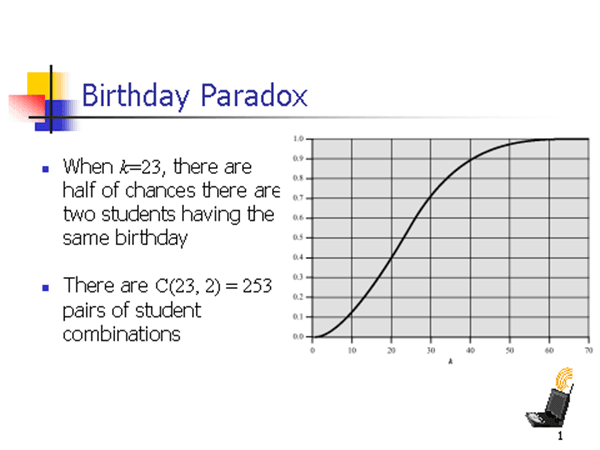The Fibonacci sequence Is Seen in the Number 1/89: The Fibonacci sequence is an amazing wonder in Maths. It’s a series wherein each number comprises a sum of the preceding numbers, usually beginning from 1. It goes in the following way:

1 (starting number)

2 (1+1)

3 (2+1)

5 (3+2)0.9999 etc = 1: In mathematics, a decimal such as 0.99999 is equal to 1. When 1 is divided by 3 is 0.333 with any infinite decimal. 0.3 when multiplied by 3 is 0.(9). However, we can consider it as 1 because whenever a number is divided or multiplied by the same number, it does not change.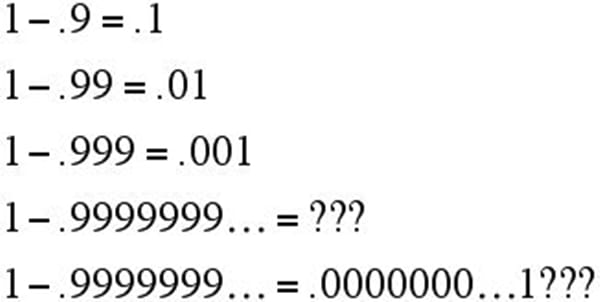Euler’s identity: Described as a wonderful equation, the Euler’s identity encompasses five neutral constants in Mathematics.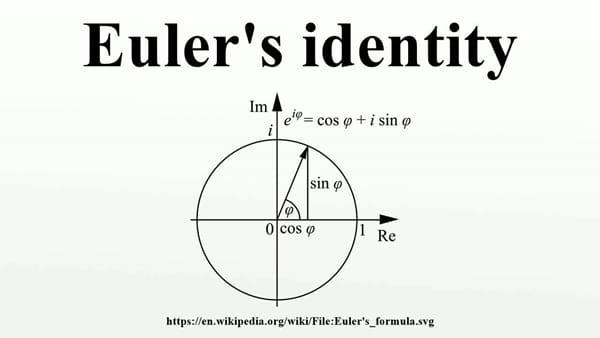Shuffling Cards: If you shuffle 52 cards in a deck, you can end up with a configuration that nobody has created. When you shuffle it properly, you can come up with a number that’s beyond astronomically large.Benford’s Law: The data like lengths of a river, heights of a building, populations in countries, you would see that the bias is towards the first digit as it is the lowest number. For instance, it is likely to be 1 than 9.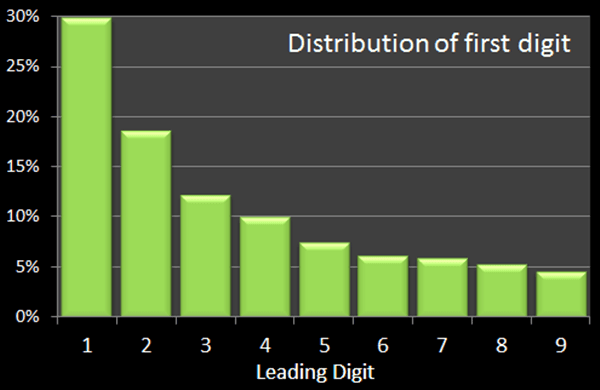Fibonacci Displays In Nature: The sequence pops up in nature. For instance, the number of petals in a Daisy flower features Fibonacci number with 34, 21, and 55 being the common one.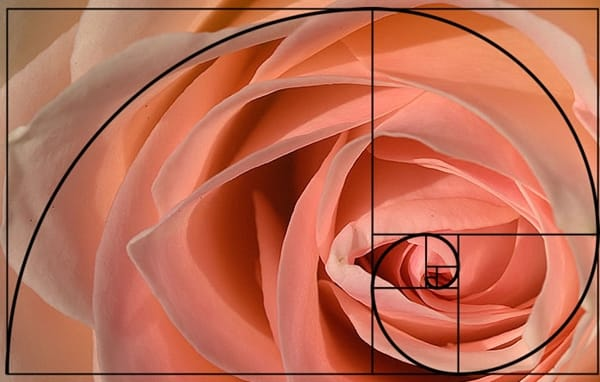111111111 * 111111111 = 12345678987654321: If you multiply numbers composed of ones, it results in a number comprising of all the figures from 1 to 9 and then back to 1. It can be written as:

111,111,111 * 111,111,111 = 12,345,678,987,654,321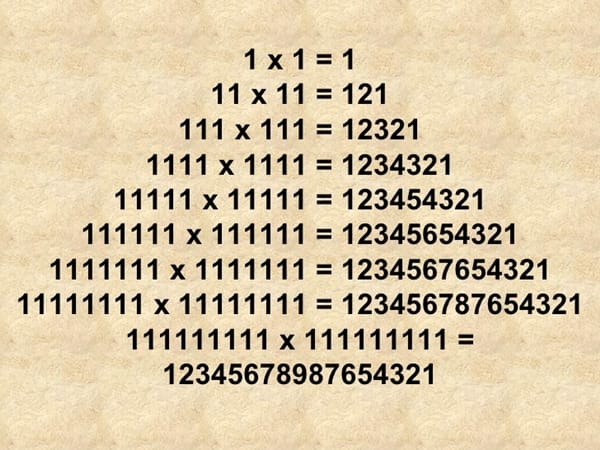Pizza Seems To Be Incredible: A Pizza with height ‘a’ and radius ‘z’ has a volume Pi × z × z × a.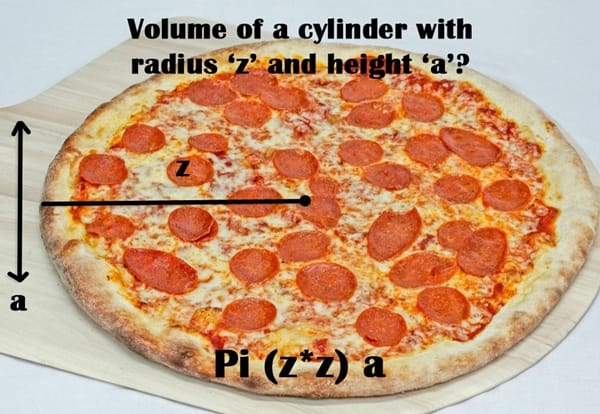Mandelbrot Set: The set is basically a collection of numbers that you can put into a simple formula (z -> z² + c) and they do not reach infinity.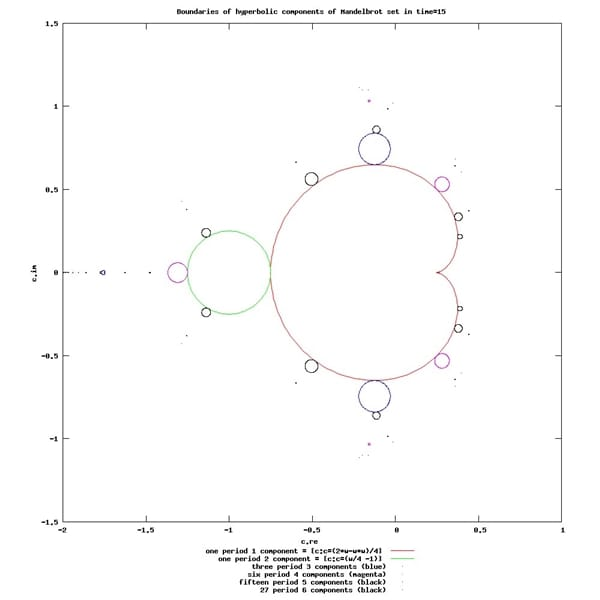Kaprekar’s constant: Kaprekar’s constant is 6174. Try this: Imagine a 4 digit number with atleast two different digits. Now put them in descending order and then in ascending order. This gives you two different numbers. Subtract the smaller from the bigger number. Then repeat the same process by taking the output as the new number. If you continuously do that, you would get Kaprekar’s constant: 6174. In fact, rep-digits like 1111, 2222 and so on are the number for which Karpekar’s constant law doesn’t work.Banach-Tarski Paradox: This signifies that you can separate one shape into two identical copies of itself.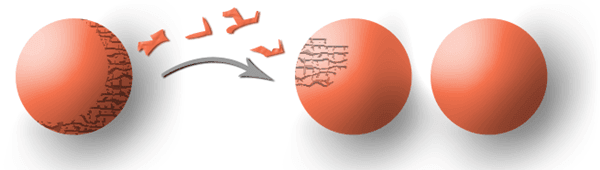Convert Miles Into Kilometers With Fibonnaci Sequence: With the help of Fibonnaci sequence, miles can be converted into kilometers.

1 1 2 3 5 8 13 21 34….

Every digit, after a few is miles whereas the number after it is close to the corresponding number of kilometer.The Decimal Fractions Of Seven Are Six Repeating Numbers: It’s an amazing daily life math fact that will blow your mind. The decimal fractions of seven results in the same six recurring digits, that too, in the same order. However, starts from different one.

1/7 = 0.142857142857…

2/7 = 0.285714285714…

3/7 = 0.428571428571…

4/7 = 0.571428571428…

5/7 = 0.714285714285…

6/7 = 0.857142857142…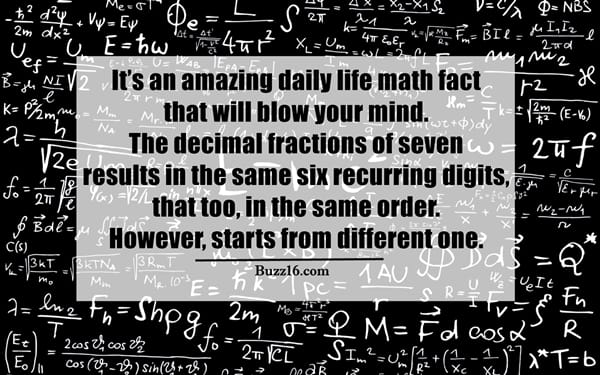Gabriel’s Horn and the Painter’s Paradox: This amazing math fact sounds familiar to calculus students. The Gabriel horn features a shape with finite volume but with infinite surface area.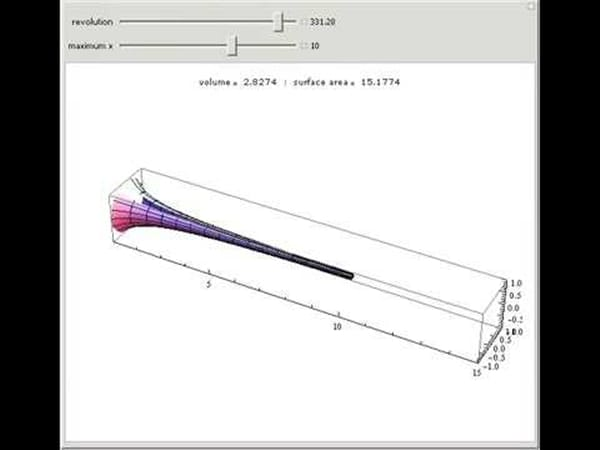Basel Problem: This theory states that if the reciprocal of all the square numbers is taken and added them together, the result is pi squared over six.Fermat’s Last Theorem: The Pythagorean theorem states that in a right triangle, a² + b² = c². Assume that all the variables are integers. Hence, the solution a=3, b=4, c=5 is permitted, however, a=1.5, b=2, c=2.5 is not allowed, though it fits the equation.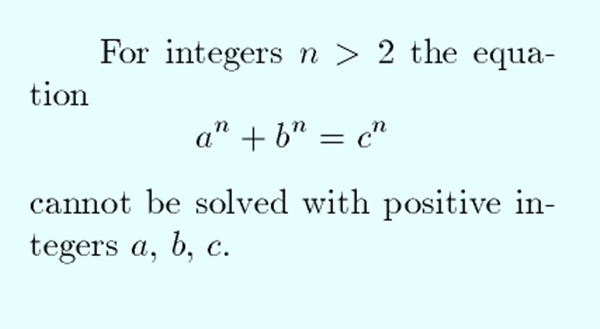Levels Of Infinity Are Different: Yes, it is true that some infinities are larger than others. In technical terms, infinity features a property that is termed as cardinality. An infinity that has a larger cardinality than the other is the larger.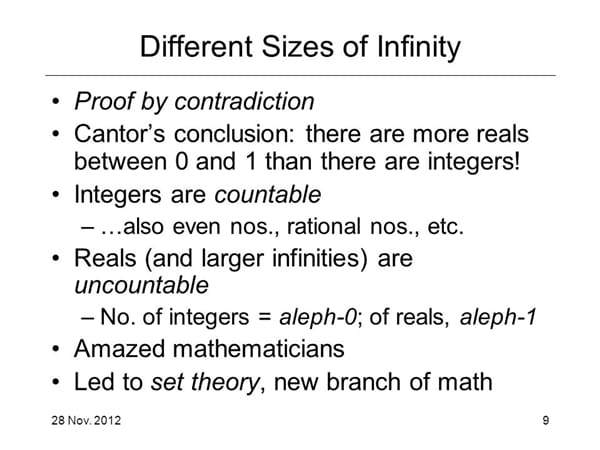26 Is The Only Natural Number: 26 is the only natural number that can be placed between a perfect cube and a perfect square.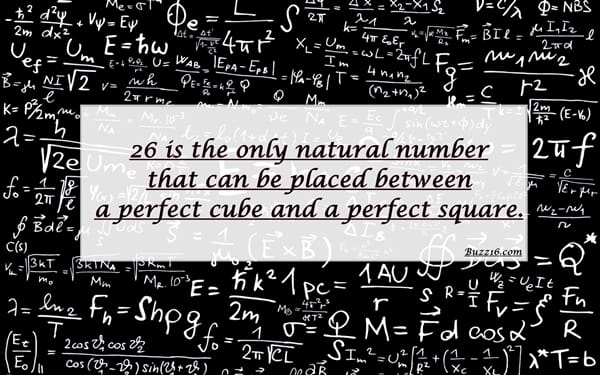It’s Impossible To Fold A Paper Seven Times: You cannot fold a paper seven times. There’s a simple calculation behind. When you fold a sheet of paper, the height gets doubled up. If you try to fold it 104 times, the height becomes more than 93 billion light years. Hence, it is believed that a paper cannot be folded 7 times.Definitely, the above daily life math facts will blow your mind and give you goose bumps.